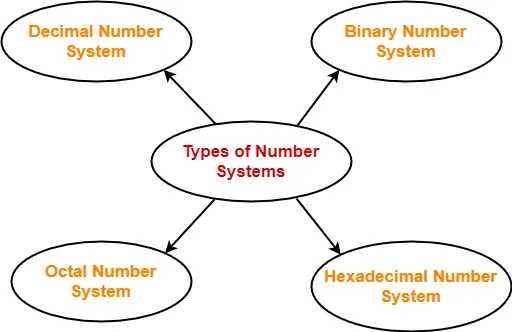## Base of Number System-

• Base of a number system is the total number of digits used in that number system.
• Number system with base ‘b’ has its digits in the range [0 , b-1].
• It is also called as radix of a number system.

## Examples-

Consider the following examples-

### Base 10 Number System-

Consider base 10 number system popularly called as decimal number system-

• Total number of digits used in this number system are 10 since it has base 10.
• These digits are 0 , 1 , 2 , 3 , 4 , 5 , 6 , 7 , 8 , 9.
• These digits clearly lie in the range [0 , base-1] = [0 , 9].

### Base 2 Number System-

Consider base 2 number system popularly called as binary number system-

• Total number of digits used in this number system are 2 since it has base 2.
• These digits are 0 and 1.
• These digits clearly lie in the range [0 , base-1] = [0 , 1].

## Important Note-

It is important to note that-

• All the digits of any number system with base ‘b’ are always less than ‘b’.
• This is clear from the range [0 , base-1] in which the digits of any number system lie.

## Types of Number System-

Four most commonly used number systems are-1. Decimal Number System
2. Binary Number System
3. Octal Number System

The following table shows the base and digits used in these number systems-

 Number System Base Digits Used Decimal Number System 10 0, 1, 2, 3, 4, 5, 6, 7, 8, 9(Total 10 digits) Binary Number System 2 0, 1(Total 2 digits) Octal Number System 8 0, 1, 2, 3, 4, 5, 6, 7(Total 8 digits) Hexadecimal Number System 16 0, 1, 2, 3, 4, 5, 6, 7, 8, 9, A, B, C, D, E, F(Total 16 digits)

To gain better understanding about Basics of Number System,

Watch this Video Lecture

Next Article- Converting Any Base to Base 10

Get more notes and other study material of Number System.

Watch video lectures by visiting our YouTube channel LearnVidFun.